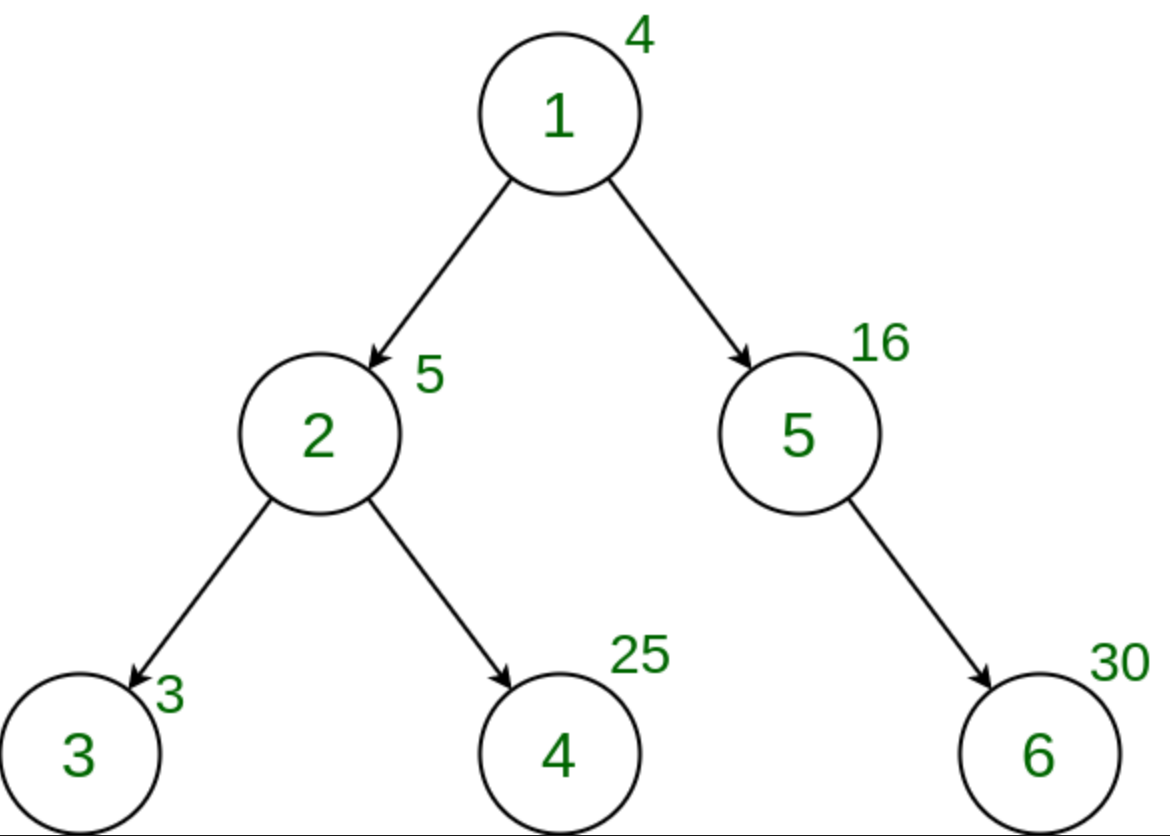# Count the nodes in the given Tree whose weight is a Perfect Number

Given a tree, and the weights of all the nodes, the task is to count the number of nodes whose weight is a Perfect number.

A perfect number is a positive integer that is equal to the sum of its proper divisors.

Examples:

Input:Output: 0
Explanation:
There is no node with a weight that is a perfect number.

## Recommended: Please try your approach on {IDE} first, before moving on to the solution.

Approach:
In order to solve this problem, we perform Depth First Search(DFS) Traversal on the tree and for every node, check if its weight is a Perfect Number or not. We keep on incrementing the counter every time such a weight is obtained. The final value of that counter after the completion of the entire tree traversal is the answer.

Below is the implementation of the above approach:

## C++

 `// C++ implementation to Count the nodes in the ` `// given tree whose weight is a Perfect Number ` ` `  `#include ` `using` `namespace` `std; ` ` `  `int` `ans = 0; ` `vector<``int``> graph; ` `vector<``int``> weight(100); ` ` `  `// Function that returns true if n is perfect ` `bool` `isPerfect(``long` `long` `int` `n) ` `{ ` `    ``// Variable to store sum of divisors ` `    ``long` `long` `int` `sum = 1; ` ` `  `    ``// Find all divisors and add them ` `    ``for` `(``long` `long` `int` `i = 2; i * i <= n; i++) { ` `        ``if` `(n % i == 0) { ` `            ``if` `(i * i != n) ` `                ``sum = sum + i + n / i; ` `            ``else` `                ``sum = sum + i; ` `        ``} ` `    ``} ` ` `  `    ``// Check if sum of divisors is equal to ` `    ``// n, then n is a perfect number ` `    ``if` `(sum == n && n != 1) ` `        ``return` `true``; ` ` `  `    ``return` `false``; ` `} ` ` `  `// Function to perform dfs ` `void` `dfs(``int` `node, ``int` `parent) ` `{ ` ` `  `    ``// If weight of the current node ` `    ``// is a perfect number ` `    ``if` `(isPerfect(weight[node])) ` `        ``ans += 1; ` ` `  `    ``for` `(``int` `to : graph[node]) { ` `        ``if` `(to == parent) ` `            ``continue``; ` `        ``dfs(to, node); ` `    ``} ` `} ` ` `  `// Driver code ` `int` `main() ` `{ ` ` `  `    ``// Weights of the node ` `    ``weight = 5; ` `    ``weight = 10; ` `    ``weight = 11; ` `    ``weight = 8; ` `    ``weight = 6; ` ` `  `    ``// Edges of the tree ` `    ``graph.push_back(2); ` `    ``graph.push_back(3); ` `    ``graph.push_back(4); ` `    ``graph.push_back(5); ` ` `  `    ``dfs(1, 1); ` `    ``cout << ans; ` ` `  `    ``return` `0; ` `} `

## Java

 `// Java implementation to Count the nodes in the ` `// given tree whose weight is a Perfect Number ` ` `  `import` `java.util.*; ` ` `  `class` `GFG{ ` ` `  `static` `int` `ans = ``0``; ` `static` `Vector []graph = ``new` `Vector[``100``]; ` `static` `int` `[]weight = ``new` `int``[``100``]; ` ` `  `// Function that returns true if n is perfect ` `static` `boolean` `isPerfect(``int` `n) ` `{ ` `    ``// Variable to store sum of divisors ` `    ``int` `sum = ``1``; ` ` `  `    ``// Find all divisors and add them ` `    ``for` `(``int` `i = ``2``; i * i <= n; i++) { ` `        ``if` `(n % i == ``0``) { ` `            ``if` `(i * i != n) ` `                ``sum = sum + i + n / i; ` `            ``else` `                ``sum = sum + i; ` `        ``} ` `    ``} ` ` `  `    ``// Check if sum of divisors is equal to ` `    ``// n, then n is a perfect number ` `    ``if` `(sum == n && n != ``1``) ` `        ``return` `true``; ` ` `  `    ``return` `false``; ` `} ` ` `  `// Function to perform dfs ` `static` `void` `dfs(``int` `node, ``int` `parent) ` `{ ` ` `  `    ``// If weight of the current node ` `    ``// is a perfect number ` `    ``if` `(isPerfect(weight[node])) ` `        ``ans += ``1``; ` ` `  `    ``for` `(``int` `to : graph[node]) { ` `        ``if` `(to == parent) ` `            ``continue``; ` `        ``dfs(to, node); ` `    ``} ` `} ` ` `  `// Driver code ` `public` `static` `void` `main(String[] args) ` `{ ` ` `  `    ``for` `(``int` `i = ``0``; i < graph.length; i++) ` `        ``graph[i] = ``new` `Vector(); ` `         `  `    ``// Weights of the node ` `    ``weight[``1``] = ``5``; ` `    ``weight[``2``] = ``10``; ` `    ``weight[``3``] = ``11``; ` `    ``weight[``4``] = ``8``; ` `    ``weight[``5``] = ``6``; ` ` `  `    ``// Edges of the tree ` `    ``graph[``1``].add(``2``); ` `    ``graph[``2``].add(``3``); ` `    ``graph[``2``].add(``4``); ` `    ``graph[``1``].add(``5``); ` ` `  `    ``dfs(``1``, ``1``); ` `    ``System.out.print(ans); ` ` `  `} ` `} ` ` `  `// This code contributed by Princi Singh `

Output:

```1
```My Personal Notes arrow_drop_upCheck out this Author's contributed articles.

If you like GeeksforGeeks and would like to contribute, you can also write an article using contribute.geeksforgeeks.org or mail your article to contribute@geeksforgeeks.org. See your article appearing on the GeeksforGeeks main page and help other Geeks.

Please Improve this article if you find anything incorrect by clicking on the "Improve Article" button below.

Improved By : princi singh